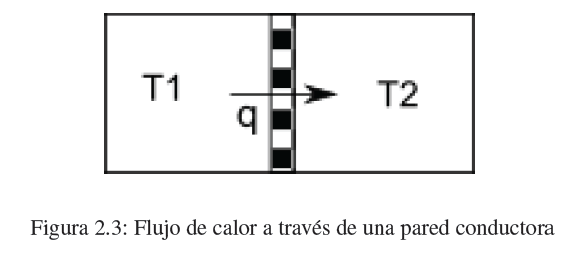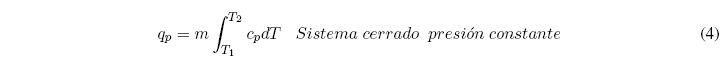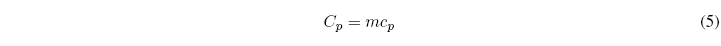Heat is the second form of energy transfer that we are going to study. When two bodies at different temperatures come into contact through a thermally conductive wall, a flow of heat is produced from the hot body to the cold one until the temperatures are equal.The amount of heat required to raise the temperature of the system by one dT is given by the expression:where m is the mass of the system and c p its specific heat at constant pressure.

The heat necessary to change the temperature of a body from T 1 to T 2 is given by the sum of the infinite infinitesimal changes necessary to go from one temperature to another. The sum of these changes is that of the following integral:Heat capacity is defined as the product of mass times specific heat.